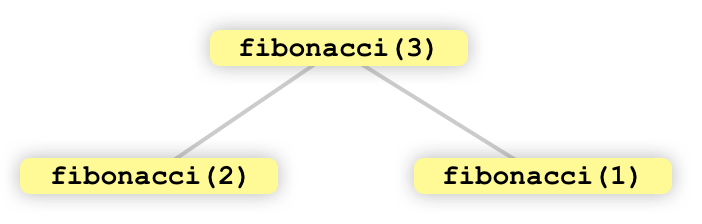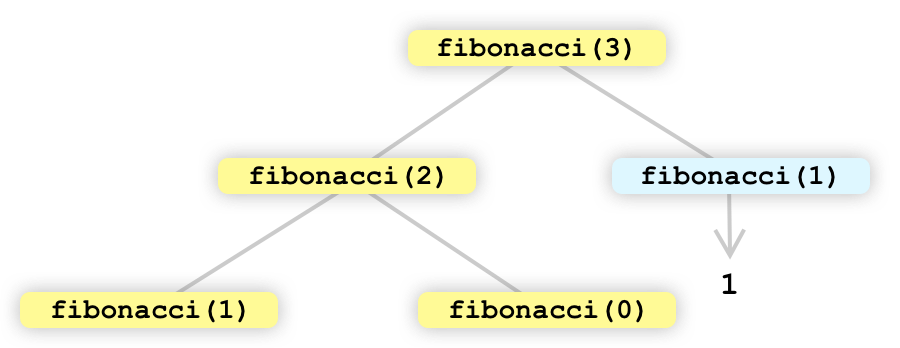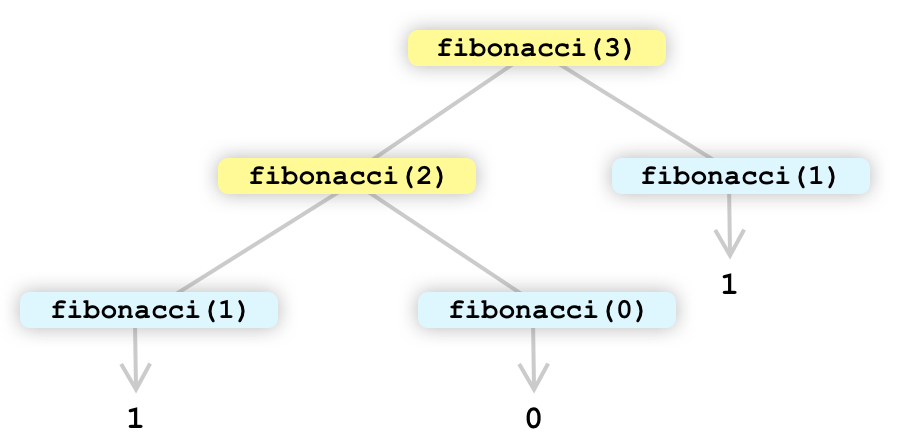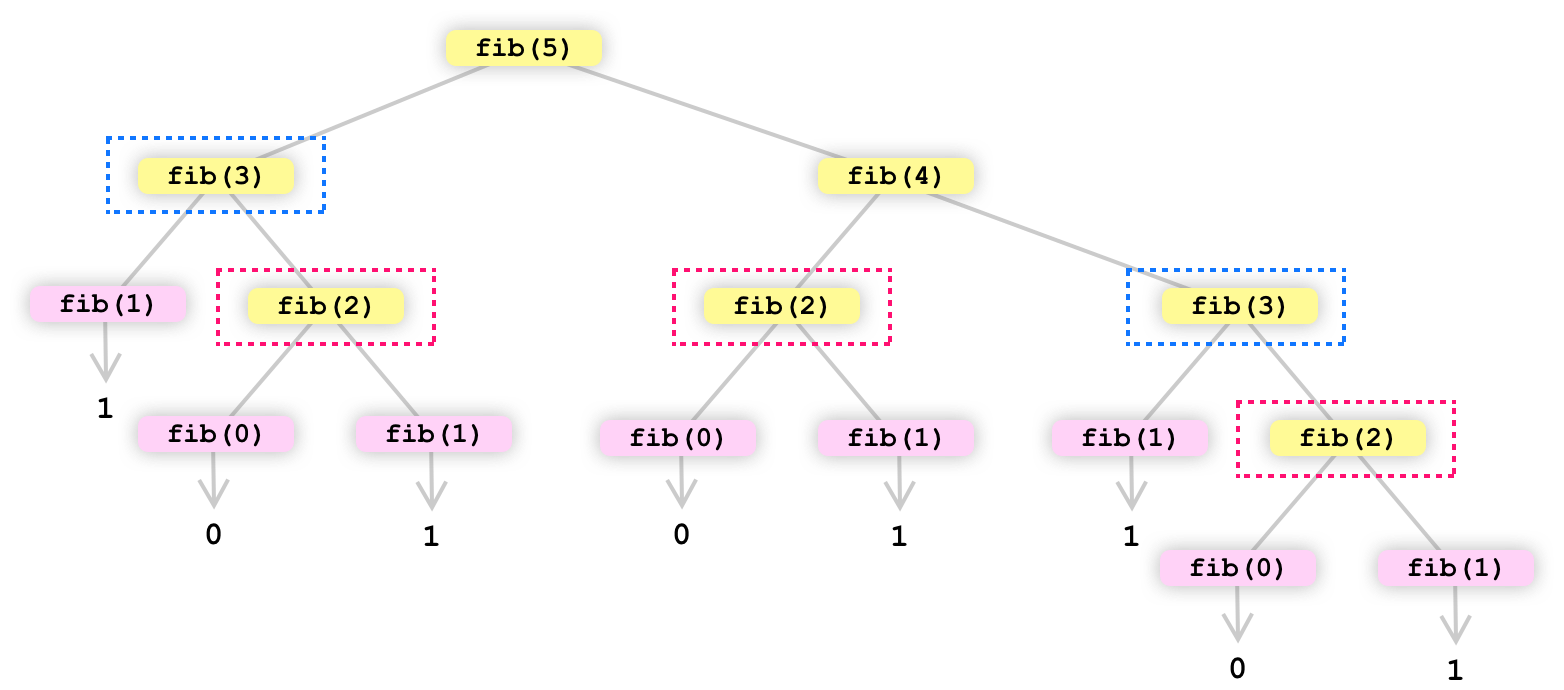Color

Background

Done

# Fibonacci and Going Beyond Recursion

by kirupa   |   filed under Data Structures and Algorithms

If there was a Greatest Hits list of popular algorithms, the Fibonacci sequence would be right at the top. It would be the Beatles or the Rolling Stones of its generation. The Fibonacci sequence is a series of numbers in which each number is the sum of the previous two numbers. The sequence starts with 0 and 1, and then each subsequent number is the sum of the previous two. So the sequence goes 0, 1, 1, 2, 3, 5, 8, 13, 21, 34, and so on.

To dive into that a bit deeper, here is how the sequence is calculated:

``````fibonacci(0) = 0
fibonacci(1) = 1
fibonacci(2) = 1 // Sum of fibonacci(1) + fibonacci(0)
fibonacci(3) = 2 // Sum of fibonacci(2) + fibonacci(1)
fibonacci(4) = 3 // Sum of fibonacci(3) + fibonacci(2)
.
.
.
fibonacci(n) = fibonacci(n-1) + fibonacci(n-2)``````

This is cool...sort of. Why do we care about it? Besides its many practical uses, the Fibonacci sequence is a great example of an algorithm that can be solved recursively:The Fibonacci sequence is also a great example of an algorithm that highlights the limitations of using recursion:It provides the perfect jumping-off point for us to learn about alternate (non-recursive!) ways to calculate the Fibonacci sequence and apply what we know to other types of computation problems that we'll encounter in the future.

Onwards!

## Recursively Solving the Fibonacci Sequence

As we saw earlier, a number in the Fibonacci sequence is the sum of the two preceding numbers. We know the first two numbers are always 0 and 1. All subsequent numbers can be calculated by using the following formula:

``fibonacci(n) = fibonacci(n-1) + fibonacci(n-2)``

If we turn all of this into JavaScript, here is a recursive way to identify for any number in the Fibonacci sequence:

``````function fibonacci(n) {
if (n == 0) {
return 0;
} else if (n == 1) {
return 1;
} else {
return fibonacci(n - 1) + fibonacci(n - 2);
}
}

console.log('Result is', fibonacci(10));``````

This function takes in a number n and returns the nth number in the Fibonacci sequence. The function works recursively by calling itself repeatedly with smaller and smaller values of n until it reaches one of the terminating conditions (where n is 0 or 1).

For example, if we call fibonacci(3), the function will first call itself with n equal to 2, and then with n equal to 1:This leads to the next step, where fibonacci(1) hits our terminating condition and returns a 1. The fibonacci(2) call will expand further:At this stage, both fibonacci(1) and fibonacci(0) will hit their respective terminating conditions and return 0 and 1, respectively:In our code, what we are doing is adding the result of each recursive call:

``````function fibonacci(n) {
if (n == 0) {
return 0;
} else if (n == 1) {
return 1;
} else {
return fibonacci(n - 1) + fibonacci(n - 2);
}
}``````

What this means is that we end up adding 1 + 0 + 1, which is 2. The 3rd number in the fibonacci sequence is indeed 2, so we did good here!

Now, we went a little lenghty in our visual explanation here, but let’s speed things up and look at one more example of us calculating the Fibonacci sequence recursively. This time, let’s calculate what the 5th number in the Fibonacci sequence will look like:If we add up the final numbers that appear, we get a value of 5 - which is exactly what the 5th number in the Fibonacci sequence actually is.

One thing to notice is the sheer number of recursive calls we are dealing with here. We aren’t even dealing with large numbers. The jump in recursive calls between us calculating the 3rd number in the Fibonacci sequence to the 5th number in the Fibonacci sequence is huge. To see how many recursive function calls our favorite Fibonacci number requires, take a look at the following chart:Your eyes aren’t deceiving you. To calculate, for example, the 20th number in the Fibonacci sequence, there are 21,891 function calls. Just four numbers later, the 24th number in the Fibonacci sequence is a whopping 150,049 function calls.

That’s a lot of calls, and each function call isn’t a cheap operation. The running time here is O(2^n). This puts it towards the high end of how expensive an operation we are performing here. There are faster ways that improve upon the recursive approach we are taking, and we’ll look into them next.

#### Why are function calls expensive?

Function calls, especially in long-running recursive operations, can be expensive (aka take a long time to run, take up a lot of memory, or both) for a few reasons:

1. Function calls require additional memory: When a function is called, the interpreter needs to store the current state of the program (including the values of all the variables) on the call stack. This can consume a lot of memory, especially if the recursion goes deep.

2. Function calls require additional processing time: Each function call requires the interpreter to push the current state onto the call stack and then pop it off again when the function returns. This can be time-consuming, especially if the function calls itself multiple times.

3. Function calls can cause stack overflow errors: If the recursion goes too deep (for example, if the function calls itself a whole bunch of times in short succession), it can cause the call stack to overflow, which can lead to a runtime error.

The more we can reduce the quantity of function calls, the faster our code will run. In the next few sections, we are going to take our recursive-only approach for calculating the Fibonacci sequence and look at ways we can greatly reduce the amount of recursive function calls we will need to make!

## Recursion with Memoization

One of the big reasons our current recursive approach is expensive is that it does a lot of duplicate work. In many of the paths our recursive call takes, we are re-calculating the result for an operation even though we may have already calculated it earlier. The following visual of the function call tree for fibonacci(5) calls out the number of duplicate calculations we perform:Ignoring the numerous fib(1) and fib(0) calls, if we had a way of remembering the result of fib(3) or fib(2) from earlier calculations, we can eliminate entire branches of duplicated work.

The technique we can use here is known as memoization. Boring definition time: memoization is a programming technique that involves storing the results of expensive function calls so that they can be reused later. To put it differently, it's a way of optimizing a function by reducing the number of times it needs to be called.

If we applied memoization to our Fibonacci sequence problem, our code can be modified as follows:

``````function fibonacci(index, cache = []) {
if (cache[index]) {
return cache[index];
}
else {
if (index <= 2) {
return 1;
} else {
cache[index] = fibonacci(index - 1, cache) +
fibonacci(index - 2, cache);
}
}
return cache[index];
}

console.log('Result is', fibonacci(10));``````

Notice that we have an array called cache that stores the result of each calculation we make. Each time we are about to make a recursive call, we check to see if we already have a result stored in our cache array:

``````function fibonacci(index, cache = []) {
if (cache[index]) {
return cache[index];
}
else {
if (index <= 2) {
return 1;
} else {
cache[index] = fibonacci(index - 1, cache) +
fibonacci(index - 2, cache);
}
}
return cache[index];
}

console.log('Result is', fibonacci(10));``````

If we don’t have the result stored, we do the full recursive call but store the result for future use:

``````function fibonacci(index, cache = []) {
if (cache[index]) {
return cache[index];
}
else {
if (index <= 2) {
return 1;
} else {
cache[index] = fibonacci(index - 1, cache) +
fibonacci(index - 2, cache);
}
}
return cache[index];
}

console.log('Result is', fibonacci(10));``````

By relying on memoization, where we store the result of calculations we have already made, we greatly reduce the number of unnecessary work our code does. We saw earlier that calculating the 20th Fibonacci sequence resulted in 13,529 calls in the purely recursive approach. By combining the recursive approach with memoization, we made a total of just 37 calls. That’s a whopping 99.7% reduction in the amount of work we are doing.

## Taking an Iteration-based Approach

The final (and fastest!) approach we will look at is one where we wave goodbye to recursion completely and take an iteration-based approach. In an iteration-based approach, we typically use a loop, such as a for loop or a while loop. Applying this to our Fibonacci sequence problem, there are a few reasons why we might choose to use an iterative approach:

1. Fast: Iterative approaches are generally faster than recursive approaches, especially for large values of n. This is because an iterative approach doesn't have to build up a stack of function calls, which can consume a lot of memory and slow down the program.
2. Simple: Iterative approaches are often simpler to understand and implement than recursive approaches, especially for beginners.
3. Clear: Iterative approaches can be easier to read and understand than recursive approaches, especially for complex problems.

Below is an example of how we could write an iterative version of the Fibonacci function we have been seeing so far:

``````function fibonacci(n) {
if (n == 0) {
return 0;
} else if (n == 1) {
return 1;
} else {
let a = 0;
let b = 1;
for (let i = 2; i <= n; i++) {
let c = a + b;
a = b;
b = c;
}
return b;
}
}

console.log('Result is', fibonacci(10));``````

As we can see, this version of the function uses a loop to iterate through the numbers in the sequence and compute the next number in the series using the previous two. It doesn't use recursion at all, and the fibonacci function is called just once.

## Going Deeper on the Speed

From what we have seen so far, the fastest approach for calculating the Fibonacci sequences is the iterative approach. The second fastest will be recursion with memoization. The slowest approach is the recursive-only one we started all this off with. Let’s get more precise. How fast is each of the approaches? Take a look at the following graph that plots the time (in milliseconds) it takes to calculate the Fibonacci sequence from 0 to 30:What you are seeing here isn’t a glitch. The time for calculating the Fibonacci sequence for the first 30 numbers is almost 0 in the recursive + memoization and iteration-based approaches. The purely recursive approach starts to take increasing amounts of time at around the 17th Fibonacci number, and it grows exponentially from there on out. There is a reason why the chart only included the first 30 numbers of the Fibonacci sequence. The recursively only approach couldn’t handle larger numbers without massive delays and ultimately leading to a stack overflow error.

If we ignore the recursive-only approach and focus our attention on the memoization and iteration approaches, here is the time for calculating the Fibonacci sequence for the first 300 (!!!) numbers:Here, we can see our iteration-based approach being a much faster solution when we compare it to the recursive approach with memoization. The reason is that, no matter how effective our memoization strategy is, recursive function calls are expensive operations. If we can avoid them entirely, like we are doing in the iteration-based approach, we will get the best results.

## Conclusion

We covered a huge boatload of ground here. The Fibonacci sequence is an important concept in computer science (and family dinner conversations involving algorithms!) because it illustrates the basic idea of recursion, which is a technique where a function calls itself to solve a problem. The problem with recursion is that it can be slow. This is especially true when we are dealing with large inputs or complex calculations that result in many recursive function calls. To address this shortcoming, we looked at two additional approaches that greatly sped up our Fibonacci calculations:

• Recursive with memoization: This involves storing the results of expensive function calls so that they can be reused later.
• Iterative: This involves using a loop to iterate through the numbers in the sequence and compute the next number in the series using the previous two.

While we looked at all of this in the context of calculating a number in the Fibonacci sequence, the concepts we saw here will continue to carry over into other problems we'll be seeing in the future. It's going to be a fun ride!

Just a final word before we wrap up. If you have a question and/or want to be part of a friendly, collaborative community of over 220k other developers like yourself, post on the forums for a quick response!